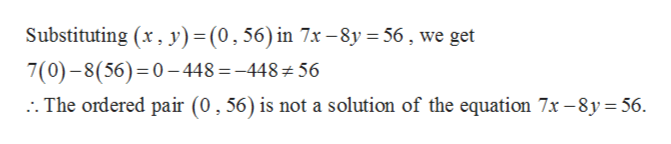# How does one determine whether an ordered pair is a solution of a given equation?Ex. 7x-8y=56; (0,56), (8,0)

Question
3 views

How does one determine whether an ordered pair is a solution of a given equation?

Ex. 7x-8y=56; (0,56), (8,0)

check_circle

Step 1

Given,

Step 2

Now

...help_outlineImage TranscriptioncloseSubstituting (x, y) = (0, 56) in 7x -8y 56, we get 7(0)-8(56) 0-448 =-448 56 The ordered pair (0,56) is not a solution of the equation 7x -8y= 56 fullscreen

### Want to see the full answer?

See Solution

#### Want to see this answer and more?

Solutions are written by subject experts who are available 24/7. Questions are typically answered within 1 hour.*

See Solution
*Response times may vary by subject and question.
Tagged in

### Equations and In-equations